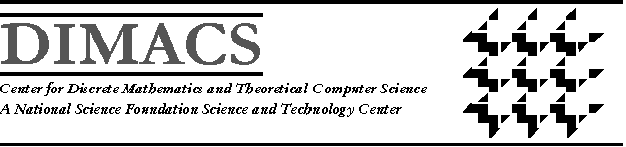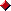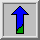######The DIMACS library of mixed semidefinite-quadratic-linear programs

#### Abstract:

To provide access to test problems for the participants of the 7th DIMACS Implementation Challenge, we assembled a library of test problems.
Our main concerns in the selection were to create a library of instances thatarise from the widest possible range of sources, and applications.are as realistic as possible.represent all levels of difficulty.have their origin and the formulation used clearly documented.
Currently, we have 12 problem sets. More are welcome; please see the "Submissions" section below.

#### Developers:Gabor Pataki University of North Carolina, Chapel HillStefan H. Schmieta Columbia University

#### Problem descriptions:A table with problem sizes and known solutions.A table listing problem originators, formulators, and donators.

#### Technical report:A preliminary version with more details.

#### Problem formats:The .mat files contain the problems in the format used by Sedumi .
that solves a problem of the form min { c'x | st. Ax=b, x in K }, where K is an appropriate cone, representing
semidefinite, quadratic, and linear constraints on x; for details, see the report.
This format is probably the easiest to convert to all other formats, if you have Matlab.
For some large graph problems, only a .dat file containing the graph description is available,
with a commented Matlab code that can generate the .mat file from it.Two converter codes are provided below, both written by Brian Borchers.

#### Reporting the solution quality:A document describing the required format for reporting the error of the obtained solutions is hereHans Mittellman's independent benchmarking results.The SDPLIB library by Brian Borchers.

#### Problem sets:The complete problem library as a tar file and compressed with gzip.The torus set:  Max cut problems from the Ising model of spin glasses.
Caveat: these max { cx | st. Ax =b, x in K } type problems
are given as min { -cx | st. Ax =b, x in K }. To get the optimal values in the table,
you must multiply the optimal value of the latter problem by (-1).
In addition, the optimal values of the torusg (that is, Gaussian instances) must be divided by 100,000.The fap set : Min k-uncut problems from frequency assignment.The bisection set : Min bisection problems from circuit partitioning.The nql set : Quadratic problems to compute plastic collapse states: plain strain models.

The problems tagged "old" contain the formulations originally present in the library. They, although equivalent, are inferior formulations and are not true to the formulations as they were submitted.The qssp set: Quadratic problems to compute plastic collapse states: supported plate models.

The problems tagged "old" contain the formulations originally present in the library. They, although equivalent, are inferior formulations and are not true to the formulations as they were submitted.The filter set: Mixed SDP/SOCP problems from PAM (pulse amplitude modulation) filter design.The hinf set: LMI (Linear Matrix Inequality) problems.The truss set: Truss topology design problemsThe antenna set: Antenna array design problemsThe copos set: Checking a sufficient condition for copositivity of a matrixThe hamming set: Instances computing the theta function of Hamming graphs for which the exact value is known.The sched set: Quadratic relaxations of scheduling problems.

The files tagged "_orig" contain the models as they were submitted. The corresponding "_scaled" files are reformulations that (among other things) scale the problem. The scale factor for the objective function is contained in the mat-file as c_mult.

#### Submissions:

To add a problem set to the collection send a description of the set to Gabor Pataki or Stefan H. Schmieta.Back to the Challenge pageBack to the DIMACS home page